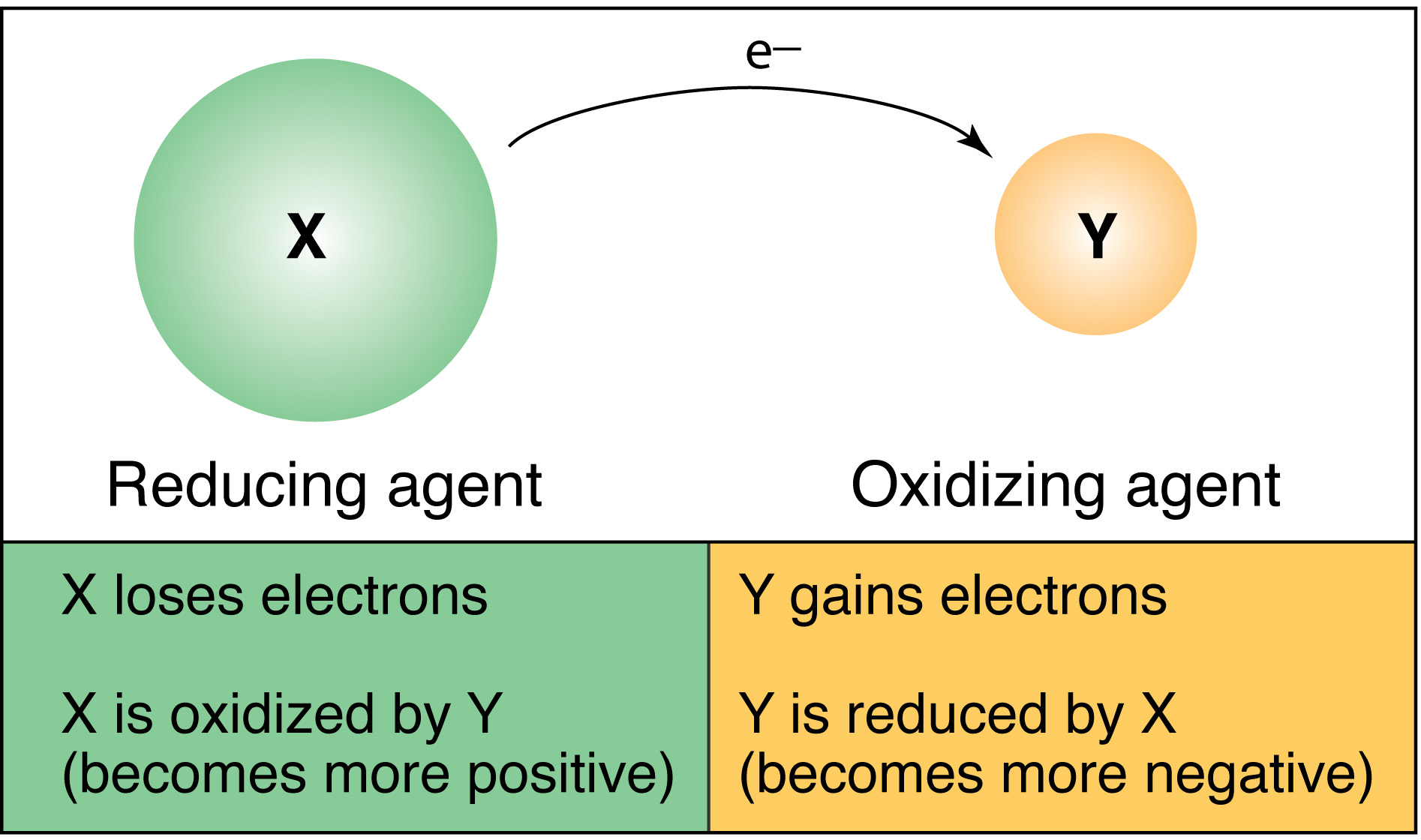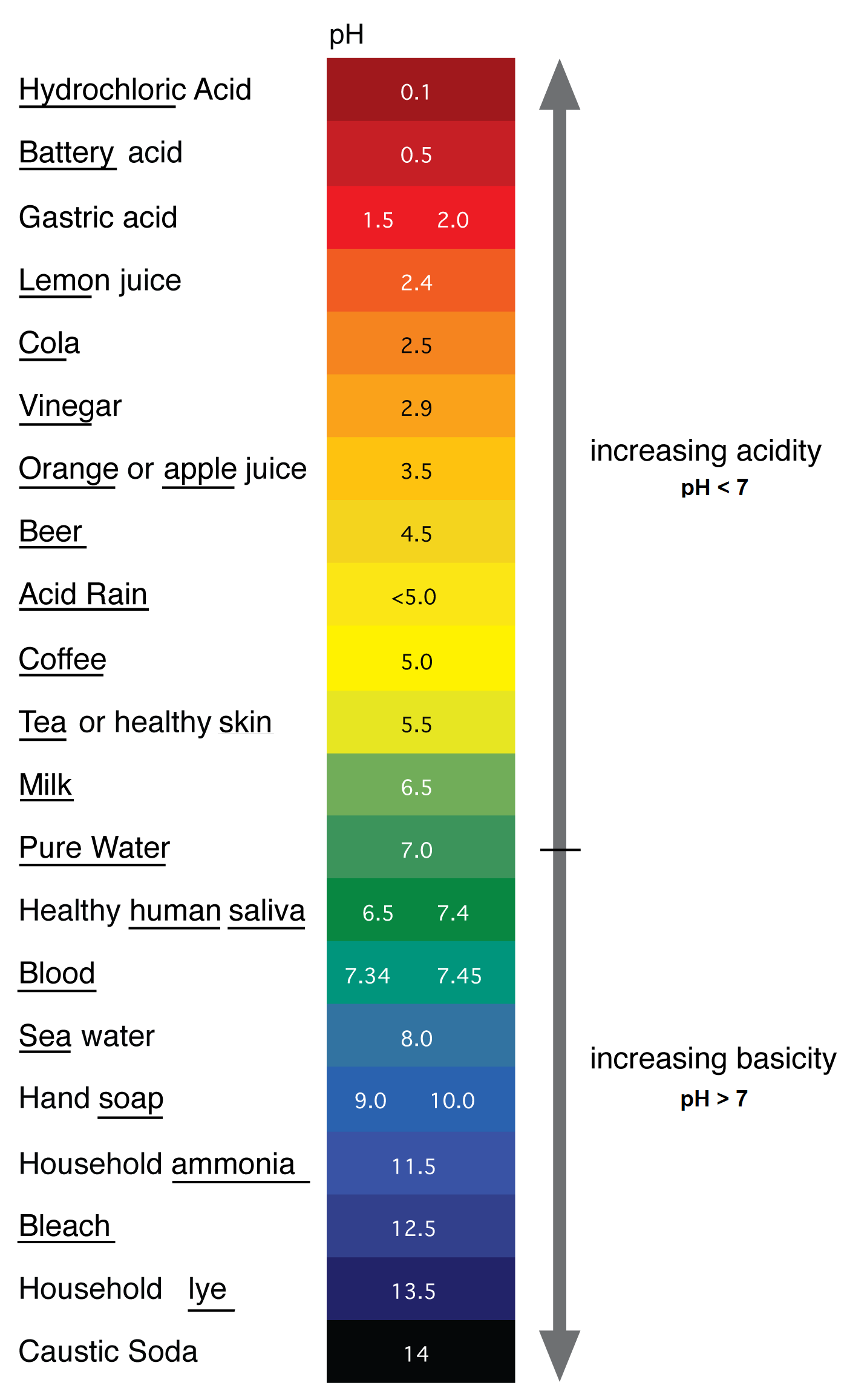Redox Chemistry Primer

A discussion of chemical principles is necessary to understand groundwater contamination problems.  A variety of parameters are measured when a sample is collected for water analysis.  The following is a short compilation and discussion of some of the more common terms and principles used in the Plume Busters discussion of chromium chemistry and groundwater.

Water is made up of hydrogen (H) and oxygen (O) atoms with a formula of: H2O.  This means that for every oxygen atom there are two hydrogen atoms bound to it.  Each of these atoms has an electrical charge associated with it, either positive or negative.  In this case the hydrogen atoms have a positive charge (H+) when not associated with oxygen and the oxygen has a negative charge (O-2).  The superscripted numbers to the right of the chemical symbol indicate the number of electrons gained or lost by chemical bonding.  A positive number indicates that the hydrogen atom gave up an electron. A negative number indicates that the oxygen atom acquired two electrons, one from each hydrogen atom, in the chemical bonding process.  The combination of atoms makes a molecule, which is bound together by the sharing of electrons with acquired electrons under the influence of the oxygen atom most of the time.  When H2O is formed the positive and negative charges are balanced out and the charge on the molecule is neutral or zero.

Water, when it dissociates, forms H+ and OH-.  A charged particle, such as (H+) or (OH-) is called an ion.  The charge, whether positive or negative, is called the oxidation number, which represents the number of electrons gained, lost, or shared in a chemical reaction.

Oxidation-Reduction Reactions

Redox reactions describe the movement of electrons from one ion to another.  The term RedOx comes from the two processes that occur during a reaction: REDuction and OXidation.  These reactions occur together, they cannot occur separately.

Oxidation is the term given to the process of gain/addition of electrons from another compound, ion, or atom.  Because the oxidizing substance removes electrons from another substance, which are then added to itself, the oxidizing substance becomes “reduced” (more negative).  And because it “accepts” electrons it is called an electron acceptor.  The oxidation number becomes more negative.

Reduction is the term given to the process of loss/removal of electrons, which are given to another compound, atom, or ion.  Because the reducing substance gives electrons to another substance it becomes “oxidized” itself (more positive).  And because it “donates” electrons it is called an electron donor.  The oxidation number becomes more positive.

The key terms involved in redox can be confusing. A mnemonic for remembering what occurs is OIL RIG: Oxidation Is Loss of electrons; Reduction Is Gain of electrons.  As an example, an element that is oxidized loses electrons and becomes more positive; however, that element is referred to as the reducing agent. If you want to know, for example, what an oxidizing agent does in terms of electrons:

• Oxidizes something else
• Oxidation is loss of electrons (OIL RIG)
• That means that an oxidizing agent takes electrons from that other substance and adds them to itself, thus the oxidation number becomes more negative.
• So an oxidizing agent must gain electrons.

In contrast, a reducing agent:

• Reduces something else
• Reduction is gain of elections (OIL RIG)
• This means that a reducing agent gives electrons to another substance and the oxidation number becomes more positive.
• A reducing agent must lose electrons

The Figure below illustrates the process of RedOx reactions.Redox reactions are evaluated the equation in terms of which ions are reduced (gain electrons) and which are oxidized (lose electrons).  This is done by writing half equations for each reaction and including the electrons in the equation.  An example of a reaction is given below for iron.

Iron forms a number of different ions for example, Fe+2 and Fe+3. If you think about how these might be produced from iron metal, the 2+ ion will be formed by oxidation of the metal by removing two electrons:

Fe+0 ® Fe+2 + 2e-

The iron is now said to be in an oxidation state of +2. Removal of another electron gives the Fe3+ ion: Fe+2 ® Fe+3 + e-.  The iron now has an oxidation state of +3.

Question:

Batteries make use of redox reactions to produce electricity. What makes this possible?

Oxidation/Reduction Potential (Eh)

Metal ions have strong and weak oxidizing/reducing potential.  In order to evaluate redox reactions, the redox potential of a solution is measured and reported as Eh.  The following site  http://www.chemguide.co.uk/physical/redoxeqia/introduction.html, shows how the Eh values are determined experimentally.  A hydrogen electrode is used as the reference to which all of the experiments are compared and has a voltage of zero.

The results are listed as voltage (or potential), which represents the driving force for “moving” the electrons from one location to another (for example, from a reducing agent to an oxidizing agent). The higher the voltage the more strongly the electrons will be moved through a solution. A positive cell potential (voltage) indicates a spontaneous electrochemical reaction. A negative cell potential (voltage) indicates a non-spontaneous reaction (the opposite reaction will, therefore, be spontaneous!).

Redox potential (voltage) is a measure of how easily a metal (or other ion) will give up electrons or retain electrons, not the likelihood for a specific oxidation or reduction reaction occurring.  The chemical equations are written as half-reactions with the most reducing reactions, donating electrons, having an Eh value that is more negative and the most oxidizing reactions, receiving electrons, having an Eh value that is the more positive.

A list of Standard electrode potentials for various reactions is given at: http://en.wikipedia.org/wiki/Standard_electrode_potential_%28data_page%29

This table illustrates that Eh values that are very negative, such as for lithium (Li, Eh = -3.04), are strong reducing agents, that is, electrons are given up quickly and the oxidation number becomes more positive.  On the other end of the chart, fluoride (F, Eh = +2.87) is a strong oxidizing agent meaning that it takes electrons and the oxidation number becomes more negative.

More negative values indicate reducing reactions occurring (loss of electrons; more positive ion results) and oxidizing reactions (gain of electrons; more negative ion results).  Higher Eh positive values suggest that reaction is more likely to occur spontaneously without the need of extra energy.

The Eh value gives an indication of how likely a given redox reaction will occur.  When the two equations are written in terms of electrons being donated or received the Eh value will indicate whether the reaction is likely to proceed.  In the case of chromium the following reactions are listed in the Standard electrode potential table referred to above:

 Cr2O72−(aq) + 14H+ + 6e− → 2Cr3+(aq) + 7H2O +1.38 volts Cr3+(aq) + 3e− → Cr(s) -0.74 volts Cr3+(aq) + e− → Cr2+(aq) -0.42 volts

Based on the voltages, the chromium(+6) (Cr2O7-2) value is a spontaneous reaction if it is in the presence of a reducing agent (Eh = +1.38 v).  The conversion of chromium(+3) to either a solid phase or to chromium(+2) is much less likely to happen based on the negative Eh potentials.

This suggests that chromium(+6) will be preferentially reduced to Chromium(+3) and then is not likely to be further transformed into a mobile form.

Redox reactions are the controlling chemistry for chromium conversion from Cr+6 to Cr +3.  Chromium (+6) is an extremely mobile form and moves rapidly with ground water.  Chromium is a very toxic chemical, especially in the Cr+6 form, to both human and environmental health.  Reduction of chromium from its Cr+6 state to Cr +3 requires a substance that will give electrons to the chromium so it will become more stable and remain in the sediment rather than move with the groundwater.

In the In Situ Redox remediation method discussed in the Remediation section of Plume Busters, a reagent is injected into the aquifer through injection/withdrawal wells at a rate and duration necessary to treat the desired volume of aquifer sediments.  This treatment volume plus the quantity of available iron in the sediments determines the quantity of reductive capacity generated in the barrier and the barrier’s productivity. The redox-altering reagent generally used is sodium dithionite (Na2S2O4).  The dithionite ion is commonly known as hydrosulfite and is a strong reducing agent, particularly in strongly basic solutions.

Questions

1. Sodium dithionite is a reducing agent. What is a reducing agent?

2. What happens when chromium (+6) is reduced?

Measuring pH

Another parameter measured in groundwater is pH.  This term means the “power of hydrogen” and is a measure of the quantity of hydrogen ions (H+) in a solution.  pH is a measure of the acidity of a solution in terms of the amount of hydrogen (H+) present (http://en.wikipedia.org/wiki/PH).  A solution has an acid pH if the value measured occurs between 0 and 7 meaning there is more H+ present.  A solution has a basic pH if the value measured occurs between 7 and 14 meaning there are more OH- ions than H+ ions present in the solution.  A neutral pH is considered 7 where there are equal numbers of H+ and OH- ions.  A ph of 7.00 is generally the value measured for uncontaminated water.

The values of pH are on a log scale and represent a ten-fold decrease in H+ ion concentration measurement as one goes up the scale.  This means that a shift in pH from 2 to 3 represents a 10-fold decrease in H+ concentration, and a shift from 2 to 4 represents a 100 (10 × 10)-fold decrease in H+ concentration.  The figure below shows a color scale of the pH values of common substances listed to the left.  One can see from the diagram that coffee is much more acidic than bleach.  This means that there are more H+ ions in coffee than in bleach.

This also means there are more OH- ions in bleach than in coffee so bleach is more basic than coffee.

The pH of groundwater is useful to help determine if contamination has occurred.  Because water generally has a pH around 6-8 a number higher or lower than that is a signal that there is something different about the water sample.  It may suggest a contamination problem if the number is a low pH (3-5) indicating the system is more acidic and potentially has more dissolved metals or contaminants.  The pH also helps to determine the type of reagents that may be needed to treat a specific contamination plume.